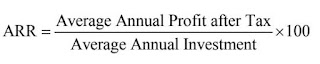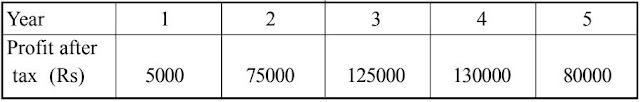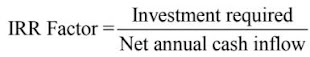We are making major changes to this site. Reach us if you are facing any issue by clicking on Report. Report

# Unit 3 Long Run Investment Decision–Capital Budgeting Focus Points only

##Unit3 Long Run Investment Decision–Capital Budgeting focus points only

The investment decision relating to the long term or fixed assets is known as capital budgeting or capital expenditure decision or long term investment decision.

The term Capital Budgeting refers to the process of decision making with regard to the investment in fixed assets (i.e., long term assets and capital projects). It involves long-term planning for proposed capital expenditure for maximizing return on investments. The capital expenditure may be;

• Cost of acquisition of fixed assets. e.g., land, building and machinery etc.
• Cost of mechanization, automation and replacement.
• Investment on research and development.
• Cost of development and expansion of existing and new projects.

### Importance of Capital Budgeting

Capital budgeting is important because of the following reasons:

1. Cost: Initial investment is huge. Hence, these decisions are planned after careful evaluation of various projects.
2. Time: The effect of the decision is known only in the near future and not immediately.
3. Irreversibility: These decisions once taken are not easily reversible without incurring huge loss.
4. Risk: The longer the time period of returns, the greater is the risk. Hence decisions should be taken after a careful review of all available information.
5. Complexity: Decisions are based on forecasting of future events and inflows. Quantification of future events involves application of statistical and probabilistic techniques.

### Process of Capital Budgeting

The process of capital budgeting decision involves five steps;1. Identify the Investment Projects
The first and crucial process of any investment decision is the recognition of opportunities. Several opportunities are available for investment but only promising opportunities that are compatible with firms objectives should be identified
2.  Evaluate the Investment Projects
It is necessary to estimate inflows and outflows of each of the investment projects. Evaluations are done by using different capital budgeting techniques.
3. Select Investment Project
After evaluation, the top management by considering returns, risk as well as the cost of capital, chooses that project which maximises the shareholders’ wealth.
4. Project Execution
Once the selection is made, the project will be implemented by acquiring necessary funds for financing the project.
5. Feedback
After the execution of the project, its progress must be monitored with the help of feedback reports. Actual performance should be compared with the expected one and deviations, if any, should be properly addressed.

### Methods of capital budgeting

Capital budgeting techniques (investment valuation criteria) can be divided into two groups:

1. Non-discounted cash flow methods (traditional methods )
1. Payback Period (PBP)
2. Accounting Rate of Return (ARR)
2. Discount cash flow methods (modern or time-adjusted methods)
1. Net Current Value (NPV)
2. Profitability Index (PI)
3. Return Rate (IRR)

1.1) Payback Period (PBP)

PBP is the number of years required to recover the original cash outlay invested in a project.

a) When the annual cash flow is constant

PBP =  Initial Investment Constant Annual Cash Inflow. If an investment of 100000 in a machine is expected to generate cash inflow of 20,000 per annum for 10 years. Calculate PBP

PBP   = Initial Investment / Constant Annual Cash Inflow

= 100000/20000 = 5 years

b) when a project’s annual cash inflows are not equal

A firm requires an initial cash outflow of 20,000 and the annual cash inflows for 5 years are 6000, 8000, 5000, 4000 and 4000 respectively. Calculate PBP.

Year      Cash Inflows      Cumulative inflows

6000                  6000

8000                  14000

5000                  19000

4000                  23000

4000                  27000

Here, When we cumulate the cash flows for the first three years, 19,000 is recovered. In the fourth year 4000 cash flow is generated by the project but we need to recover only 1000. So the time required for recovering 1000 will be ( 1000/ 4000)×12 months = 3 months. Thus, the PBP is 3 years and 3 months (3.25 years).

Decision Rule:

If the PBP is less than the maximum acceptable payback period, accept the project.
If the PBP is greater than the maximum acceptable payback period, reject the project

1. This method is simple to calculate and easy to operate.
2. It is suitable in the case of industries where the risk of technological obsolescence is very high.
3. It clarifies the concept of profit or surplus.
4. When funds are limited projects having shorter payback should be selected.
5. This method promotes liquidity by stressing on projects with earlier cash inflows.

Limitations

1. It stress on capital recovery rather than profitability.
2. 2.It does not consider post payback cash flows.
3. 3.This method ignores time value of money

1.2) Accounting/Average Rate of Return (ARR)

It is also called Return On Investment (ROI), Return On Capital Employed (ROCE)

Average Annual Profit after TaxFor example, a project requires an investment of 10,00,000. The plant & machinery required under the project will have a scrap value of 80,000 at the end of its useful life of 5 years. The profits after tax and depreciation are estimated to be as follows:Decision Rule:

• If the ARR is higher than the minimum rate established by the management, accept the project.
• If the ARR is less than the minimum rate established by the management, reject the project.

2.1) Net Present Value (NPV)

Net Present Value (NPV) is the difference between the present value of cash inflows and the present value of cash outflows.

Decision Rule:

• If the NPV is greater than 0, accept the project. .
• If the NPV is less than 0, reject the project.

1. 1.It considers the concept of time value of money.
2. 2. Unlike payback period, all cash flows are considered.

Limitations

1. It involves complex calculations in discounting and present value calculations.
2. It ignores the difference in initial cash outlays, size of different proposals etc.

Calculate NPV for a Project X initially costing Rs.250000. It has10% cost of capital. It generates following cash flows:2.2)Profitability Index (PI)

Profitability Index (PI)or Benefit-cost ratio (B/C) is similar to the NPV approach. It  can be defined as the ratio which is obtained by dividing the present value of future cash inflows by the present value of cash outlays.

PI = Total discounted Cash inflows/ Initial cash outlay

Decision Rule:

• Accept the project when PI>1 .
• Reject the project when PI<1 .
• Mayor may not accept when PI=1, the firm is indifferent to the project.

1. This method considers time value of money.
2. It is a better project evaluation technique than NPV, and helps in ranking projects where NPV is positive.

Limitation

1. It fails as a guide in resolving capital rationing problems, when projects are indivisible.

2.3) Internal Rate of Return (IRR)Internal rate of return(IRR) is the discount rate at which the net present value of an investment becomes zero. In other words, IRR is the discount rate which equates the present value of the future cash flows of an investment with the initial investment.